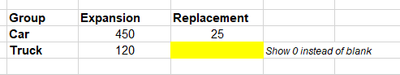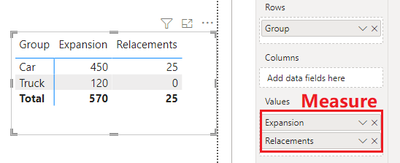cancel
Showing results for
Did you mean:Frequent Visitor

## Showing Zeros instead of Blank not working in Matrix

Hello all,

I have only been involved with Power BI/DAX for a short time.

However I am fully aware of this issue has already been discussed a couple of times also in this forum and I have of course already read the related posts, but I still did not get it to work.

Basically I have a very simple data modell (data comes from an SQL data base) with one column including different vehicle types/groups (e.g. cars) and another column indicating the corresponding quantity for the specific type. Basically the records represent different purchase requests (expansion, relacements).

What I want to achieve is a very simple matrix in power bi (similar to a pivot table in excel), in which I have the different vehicle types as rows, the two purchase requests (expansion, relacements) as columns and finally the quantity as values. So in the end I will get something like this:So far so good. But some of the groups does not contain any data for the different purchse requests, like Truck/Replacement in the expample above, which results in showing a blank cell. Instead I want zeros to be displayed in such cases, which is exactly my problem. Referring to the other posts and solutions what I have done so far are some different version of creating measures as follows, which should alternatively serve as values for the matrix:

``````Quantity Total - Display Zeros =
var SumQuantityTotal = SUM(KTA_Data[Quantity Total])
return
if(ISBLANK(SumQuantityTotal), 0 , SumQuantityTotal)``````

Referring to this post I also tried using the new Coalese-Function but still the blank value do not show as zero.

``Sum Quantity = SUM(KTA_Data[Quantity Total])``
``````Quantity Total - Display Zeros =
COALESCE([Sum Quantity], 0)``````

I would be very grateful if someone could help me here. Thank you very much in advance.

Jo

2 ACCEPTED SOLUTIONSCommunity Support

Hi @_JoSi_DA ,

This is my test table:The original matrix:``````Measure =
var sumquantity = SUM('Table'[Quantity])
return
IF(sumquantity = BLANK(),0,sumquantity)``````

The current matrix:Best regards,

If this post helps, then please consider Accept it as the solution to help the other members find it more quickly.Community Support

Hi @_JoSi_DA ,

OK, I know the reason for the problem.

This is my test table, I deleted blank rows:``````Expansion =
var sum_expansion = CALCULATE(SUM('Table'[Quantity]),FILTER('Table','Table'[Type] = "Expansion"))
return
IF(sum_expansion =BLANK(),0,sum_expansion)

Relacements =
var sum_relacements = CALCULATE(SUM('Table'[Quantity]),FILTER('Table','Table'[Type] = "Relacements"))
return
IF(sum_relacements = BLANK(),0,sum_relacements)``````

Then create a matrix like below:Please note: The fields placed in values are the measures just created, not the column in the table.

Best regards,

If this post helps, then please consider Accept it as the solution to help the other members find it more quickly.

4 REPLIES 4Frequent Visitor

I tried your DAX, but unfortunatly it still shows the blank cells.However, the difference between your test table and my data is, that I do not really have any blank cells in my table, like you have in the last 2 rows (Truck -> Replacement -> blank). So every row in my table contains a real value in the quantity column, but referring to your test table, the last two rows would not be in my table at all.

So this is why I think the IsBlank() does not work properly.

Greetings,
JohannesCommunity Support

Hi @_JoSi_DA ,

OK, I know the reason for the problem.

This is my test table, I deleted blank rows:``````Expansion =
var sum_expansion = CALCULATE(SUM('Table'[Quantity]),FILTER('Table','Table'[Type] = "Expansion"))
return
IF(sum_expansion =BLANK(),0,sum_expansion)

Relacements =
var sum_relacements = CALCULATE(SUM('Table'[Quantity]),FILTER('Table','Table'[Type] = "Relacements"))
return
IF(sum_relacements = BLANK(),0,sum_relacements)``````

Then create a matrix like below:Please note: The fields placed in values are the measures just created, not the column in the table.

Best regards,

If this post helps, then please consider Accept it as the solution to help the other members find it more quickly.Community Support

Hi @_JoSi_DA ,

This is my test table:The original matrix:``````Measure =
var sumquantity = SUM('Table'[Quantity])
return
IF(sumquantity = BLANK(),0,sumquantity)``````

The current matrix:Best regards,

If this post helps, then please consider Accept it as the solution to help the other members find it more quickly.Frequent Visitor

you are the man 😎 That did the job.
I see I will have to force myself changing my approach when working with Power BI and DAX in the future. However can not quite understand why obviously Blank() seems to work only in combination with the Calculate-Function and not already, when using 'only' the Sum-Function?!

...anyway. Thank you very much for your help

Greetings,
JohannesAnnouncements#### Exclusive opportunity for Women!

Join us for a free, hands-on Microsoft workshop led by women trainers for women where you will learn how to build a Dashboard in a Day!#### Power Platform Conference-Power BI and Fabric Sessions

Join us Oct 1 - 6 in Las Vegas for the Microsoft Power Platform Conference.Top Solution Authors
Top Kudoed Authors
Users online (1,935)